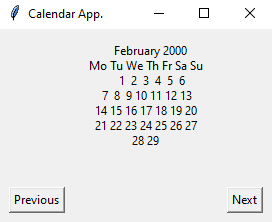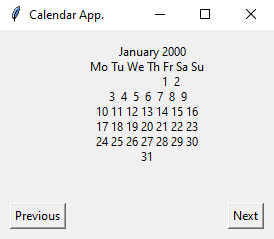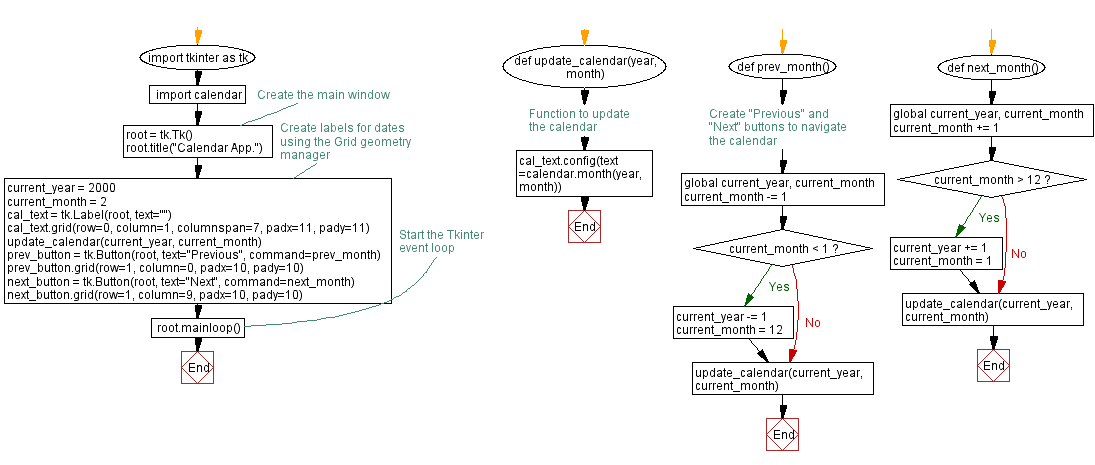﻿ Build a basic calendar application with Python Tkinter

# Create a basic calendar application with Python Tkinter

## Python tkinter layout management: Exercise-8 with Solution

Write a Python program that creates a basic calendar application with labels for days of the week and dates. Use the Grid geometry manager to arrange the labels.

Sample Solution:

Python Code:

``````import tkinter as tk
import calendar

# Function to update the calendar
def update_calendar(year, month):
cal_text.config(text=calendar.month(year, month))

# Create the main window
root = tk.Tk()
root.title("Calendar App.")

# Create labels for dates using the Grid geometry manager
current_year = 2000
current_month = 2
cal_text = tk.Label(root, text="")
update_calendar(current_year, current_month)

# Create "Previous" and "Next" buttons to navigate the calendar
def prev_month():
global current_year, current_month
current_month -= 1
if current_month < 1:
current_year -= 1
current_month = 12
update_calendar(current_year, current_month)

def next_month():
global current_year, current_month
current_month += 1
if current_month > 12:
current_year += 1
current_month = 1
update_calendar(current_year, current_month)

prev_button = tk.Button(root, text="Previous", command=prev_month)

next_button = tk.Button(root, text="Next", command=next_month)

# Start the Tkinter event loop
root.mainloop()
```
```

Sample Output:

``````
``````

Flowchart:Python Code Editor:

What is the difficulty level of this exercise?

Test your Programming skills with w3resource's quiz.

﻿

## Python: Tips of the Day

Summing a sequence of numbers (calculating the sum of zero to ten with skips):

```>>> l = range(0,10,2)
>>> sum(l)
20
```

We are closing our Disqus commenting system for some maintenanace issues. You may write to us at reach[at]yahoo[dot]com or visit us at Facebook